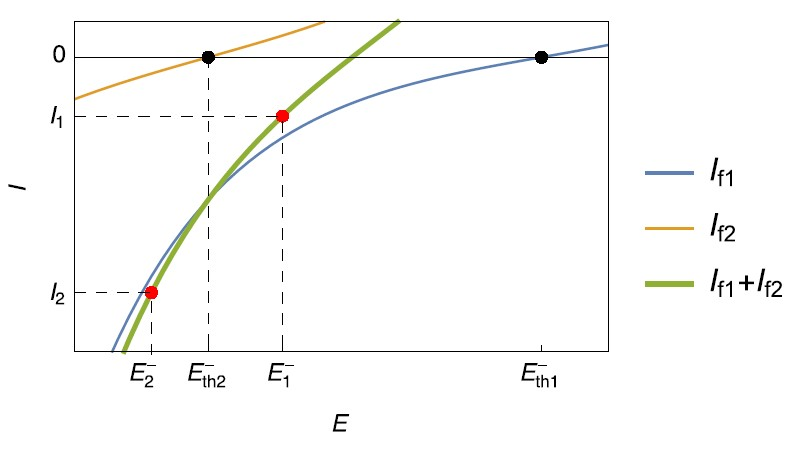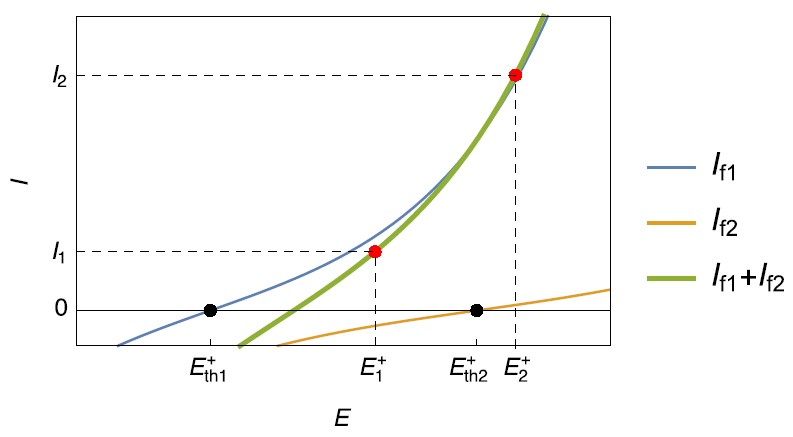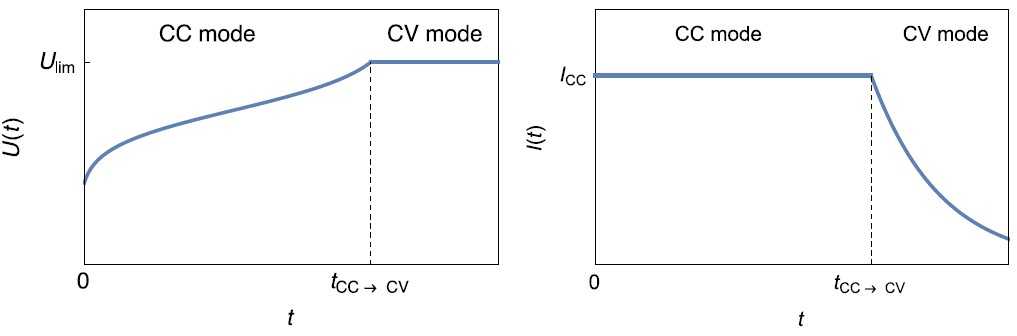Topic 5 min read# How to avoid side reactions during battery charging/discharging?

Latest updated: June 2, 2023

Introduction

The most effective way to charge a battery is by using a potentiostat / galvanostat and the CC-CV (Constant Current-Constant Voltage) protocol. While a small current value ensures the reversibility of the charging process, adding a voltage limit prevents unwanted side reactions from occurring. The following article explains why.

Battery electrodes with unwanted reactions

Let us consider the negative electrode, during the charging of a battery cell, where two concurrent faradaic reactions can take place simultaneously:

$$\mathrm{O}_1 + \mathrm{n}_1\mathrm{e}\leftrightarrow \mathrm{R}_1 \tag{1} \label{1}$$

$$\mathrm{O}_2 + \mathrm{n}_2\mathrm{e}\leftrightarrow \mathrm{R}_2 \tag{2} \label{2}$$

with two different standard potentials $E_\mathrm{th1}^- \gt E_\mathrm{th2}^-$  (Fig. 1).

For example, reaction 1 could be Li insertion and reaction 2, Li plating.Figure 1: Steady-state current vs. potential curves for simultaneous reduction reactions occurring on a negative electrode during charging.

As seen in Fig. 1, if the charge current is $I_1$, only the reaction $\eqref{1}$ occurs in the reduction direction, and the potential is maintained at $E_1^-$, a value greater than $E_\mathrm{th2}^-$ but lower than $E_\mathrm{th1}^-$. The reaction $\eqref{2}$ occurs in the oxidation reaction.

If the charge current is $I_2$, both reaction $\eqref{1}$  and reaction $\eqref{2}$ occur in the reduction direction, as the potential $E_2^-$ is lower than both $E_\mathrm{th1}^-$ and $E_\mathrm{th2}^-$. The side reaction $\eqref{2}$ in the reduction direction may be unwanted, for example Li plating.

Similarly at the positive electrode during charging, an equivalent behavior can be encountered: a second, possibly unwanted, oxidation reaction may occur when the electrode potential becomes higher than $E_\mathrm{th2}^+$ (Fig. 2) for a current $I_2$.

For a current $I_1$, the corresponding potential is $E_1^+$ and $E_\mathrm{th1}^+\lt E_1^+ \lt E_\mathrm{th2}^+$ , only the reaction $\eqref{1}$ occurs (Fig. 2) in the oxidation direction. The reaction $\eqref{2}$ occurs in the reduction direction.

The reaction $\eqref{2}$ could be, in this case, an oxygen evolution reaction.Figure 2: Steady-state current vs. potential curves for simultaneous oxidation reactions, occurring on a positive electrode during charging.

The two examples above show that unwanted reactions, that may deteriorate the battery, can be avoided during charge or discharge by preventing the electrodes from reaching a certain potential or the battery from reaching a certain voltage value. Using the constant current-constant voltage (CC-CV) charging technique, the presence of these side reactions can be avoided (Fig. 3).

The CC-CV technique

The CC-CV (Constant Current-Constant Voltage) technique consists in applying a fixed current with a voltage limit $U_\mathrm{lim}$. When this voltage limit is reached, the control mode shifts from galvano (CC) to potentio (CV) and the voltage limit is used as a set value. Choosing the right value for this voltage prevents unwanted side reactions to occur. During CV mode, the current slowly goes to 0, corresponding to a state of equilibrium in the battery.Figure 3: Left: $U(t)$ vs. $t$ curve and Right: $I(t)$ vs. $t$ during the charge of a battery using CC-CV technique.

battery charge battery discharge parasitic reactions CCCV CC-CV

## Related products

Work smarter. Not harder.

Tech-tips, theory, latest functionality, new products & more.

No thanks!